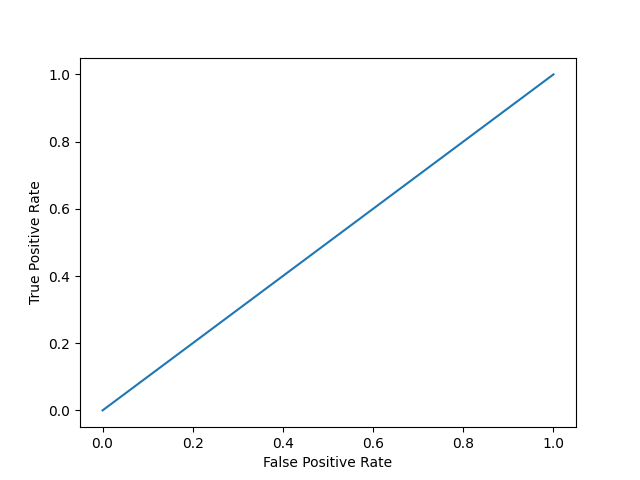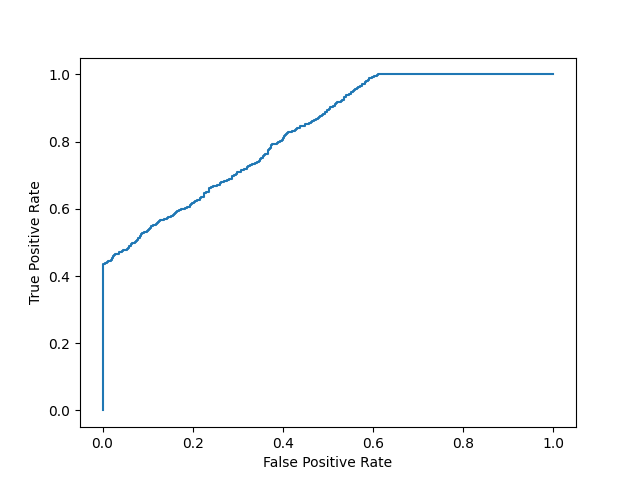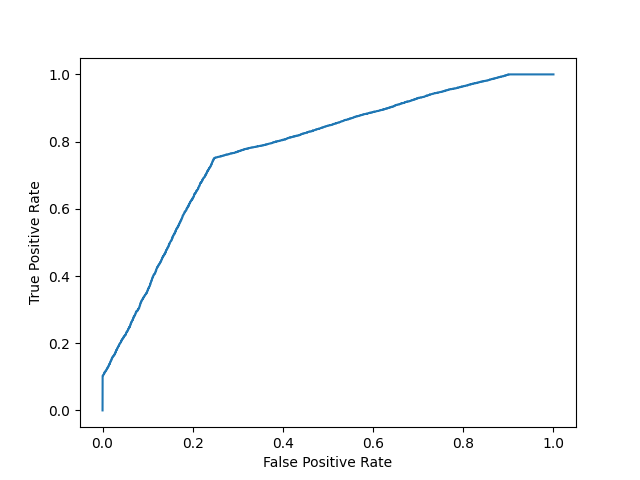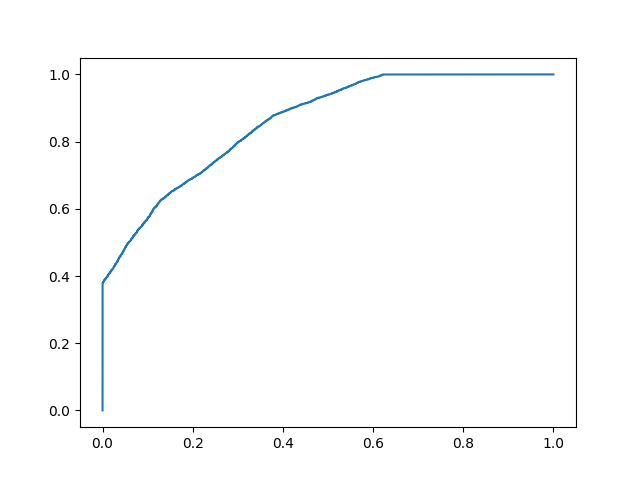## Python Tutorial

Python HOME Python Intro Python Get Started Python Syntax Python Comments Python Variables Python Data Types Python Numbers Python Casting Python Strings Python Booleans Python Operators Python Lists Python Tuples Python Sets Python Dictionaries Python If...Else Python While Loops Python For Loops Python Functions Python Lambda Python Arrays Python Classes/Objects Python Inheritance Python Iterators Python Polymorphism Python Scope Python Modules Python Dates Python Math Python JSON Python RegEx Python PIP Python Try...Except Python User Input Python String Formatting

## File Handling

Python File Handling Python Read Files Python Write/Create Files Python Delete Files

## Python Modules

NumPy Tutorial Pandas Tutorial SciPy Tutorial Django Tutorial

## Python Matplotlib

Matplotlib Intro Matplotlib Get Started Matplotlib Pyplot Matplotlib Plotting Matplotlib Markers Matplotlib Line Matplotlib Labels Matplotlib Grid Matplotlib Subplot Matplotlib Scatter Matplotlib Bars Matplotlib Histograms Matplotlib Pie Charts

## Machine Learning

Getting Started Mean Median Mode Standard Deviation Percentile Data Distribution Normal Data Distribution Scatter Plot Linear Regression Polynomial Regression Multiple Regression Scale Train/Test Decision Tree Confusion Matrix Hierarchical Clustering Logistic Regression Grid Search Categorical Data K-means Bootstrap Aggregation Cross Validation AUC - ROC Curve K-nearest neighbors

## Python MySQL

MySQL Get Started MySQL Create Database MySQL Create Table MySQL Insert MySQL Select MySQL Where MySQL Order By MySQL Delete MySQL Drop Table MySQL Update MySQL Limit MySQL Join

## Python MongoDB

MongoDB Get Started MongoDB Create Database MongoDB Create Collection MongoDB Insert MongoDB Find MongoDB Query MongoDB Sort MongoDB Delete MongoDB Drop Collection MongoDB Update MongoDB Limit

## Python Reference

Python Overview Python Built-in Functions Python String Methods Python List Methods Python Dictionary Methods Python Tuple Methods Python Set Methods Python File Methods Python Keywords Python Exceptions Python Glossary

## Module Reference

Random Module Requests Module Statistics Module Math Module cMath Module

## Python How To

Remove List Duplicates Reverse a String Add Two Numbers

## Python Examples

Python Examples Python Compiler Python Exercises Python Quiz Python Bootcamp Python Certificate

# Machine Learning - AUC - ROC Curve

On this page, W3schools.com collaborates with NYC Data Science Academy, to deliver digital training content to our students.

## AUC - ROC Curve

In classification, there are many different evaluation metrics. The most popular is accuracy, which measures how often the model is correct. This is a great metric because it is easy to understand and getting the most correct guesses is often desired. There are some cases where you might consider using another evaluation metric.

Another common metric is AUC, area under the receiver operating characteristic (ROC) curve. The Reciever operating characteristic curve plots the true positive (TP) rate versus the false positive (FP) rate at different classification thresholds. The thresholds are different probability cutoffs that separate the two classes in binary classification. It uses probability to tell us how well a model separates the classes.

## Imbalanced Data

Suppose we have an imbalanced data set where the majority of our data is of one value. We can obtain high accuracy for the model by predicting the majority class.

### Example

import numpy as np
from sklearn.metrics import accuracy_score, confusion_matrix, roc_auc_score, roc_curve

n = 10000
ratio = .95
n_0 = int((1-ratio) * n)
n_1 = int(ratio * n)

y = np.array( * n_0 +  * n_1)
# below are the probabilities obtained from a hypothetical model that always predicts the majority class
# probability of predicting class 1 is going to be 100%
y_proba = np.array(*n)
y_pred = y_proba > .5

print(f'accuracy score: {accuracy_score(y, y_pred)}')
cf_mat = confusion_matrix(y, y_pred)
print('Confusion matrix')
print(cf_mat)
print(f'class 0 accuracy: {cf_mat/n_0}')
print(f'class 1 accuracy: {cf_mat/n_1}')
Run example »

Although we obtain a very high accuracy, the model provided no information about the data so it's not useful. We accurately predict class 1 100% of the time while inaccurately predict class 0 0% of the time. At the expense of accuracy, it might be better to have a model that can somewhat separate the two classes.

### Example

# below are the probabilities obtained from a hypothetical model that doesn't always predict the mode
y_proba_2 = np.array(
np.random.uniform(0, .7, n_0).tolist() +
np.random.uniform(.3, 1, n_1).tolist()
)
y_pred_2 = y_proba_2 > .5

print(f'accuracy score: {accuracy_score(y, y_pred_2)}')
cf_mat = confusion_matrix(y, y_pred_2)
print('Confusion matrix')
print(cf_mat)
print(f'class 0 accuracy: {cf_mat/n_0}')
print(f'class 1 accuracy: {cf_mat/n_1}')
Run example »

For the second set of predictions, we do not have as high of an accuracy score as the first but the accuracy for each class is more balanced. Using accuracy as an evaluation metric we would rate the first model higher than the second even though it doesn't tell us anything about the data.

In cases like this, using another evaluation metric like AUC would be preferred.

```import matplotlib.pyplot as plt def plot_roc_curve(true_y, y_prob):     """     plots the roc curve based of the probabilities     """     fpr, tpr, thresholds = roc_curve(true_y, y_prob)     plt.plot(fpr, tpr)     plt.xlabel('False Positive Rate')     plt.ylabel('True Positive Rate')```

### Example

Model 1:

plot_roc_curve(y, y_proba)
print(f'model 1 AUC score: {roc_auc_score(y, y_proba)}')

### Resultmodel 1 AUC score: 0.5

Run example »

### Example

Model 2:

plot_roc_curve(y, y_proba_2)
print(f'model 2 AUC score: {roc_auc_score(y, y_proba_2)}')

### Resultmodel 2 AUC score: 0.8270551578947367

Run example »

An AUC score of around .5 would mean that the model is unable to make a distinction between the two classes and the curve would look like a line with a slope of 1. An AUC score closer to 1 means that the model has the ability to separate the two classes and the curve would come closer to the top left corner of the graph.

## Probabilities

Because AUC is a metric that utilizes probabilities of the class predictions, we can be more confident in a model that has a higher AUC score than one with a lower score even if they have similar accuracies.

In the data below, we have two sets of probabilites from hypothetical models. The first has probabilities that are not as "confident" when predicting the two classes (the probabilities are close to .5). The second has probabilities that are more "confident" when predicting the two classes (the probabilities are close to the extremes of 0 or 1).

### Example

import numpy as np

n = 10000
y = np.array( * n +  * n)
#
y_prob_1 = np.array(
np.random.uniform(.25, .5, n//2).tolist() +
np.random.uniform(.3, .7, n).tolist() +
np.random.uniform(.5, .75, n//2).tolist()
)
y_prob_2 = np.array(
np.random.uniform(0, .4, n//2).tolist() +
np.random.uniform(.3, .7, n).tolist() +
np.random.uniform(.6, 1, n//2).tolist()
)

print(f'model 1 accuracy score: {accuracy_score(y, y_prob_1>.5)}')
print(f'model 2 accuracy score: {accuracy_score(y, y_prob_2>.5)}')

print(f'model 1 AUC score: {roc_auc_score(y, y_prob_1)}')
print(f'model 2 AUC score: {roc_auc_score(y, y_prob_2)}')
Run example »

### Example

Plot model 1:

plot_roc_curve(y, y_prob_1)

### ResultRun example »

### Example

Plot model 2:

fpr, tpr, thresholds = roc_curve(y, y_prob_2)
plt.plot(fpr, tpr)

### ResultRun example »

Even though the accuracies for the two models are similar, the model with the higher AUC score will be more reliable because it takes into account the predicted probability. It is more likely to give you higher accuracy when predicting future data.

W3Schools is optimized for learning and training. Examples might be simplified to improve reading and learning. Tutorials, references, and examples are constantly reviewed to avoid errors, but we cannot warrant full correctness of all content. While using W3Schools, you agree to have read and accepted our terms of use, cookie and privacy policy.